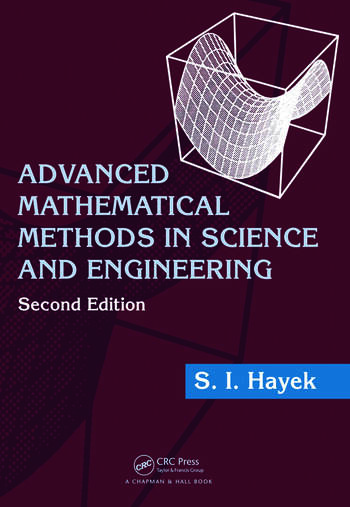Advanced Mathematical Methods in Science and Engineering

2nd Edition

S.I. Hayek

Chapman and Hall/CRC
Published June 22, 2010
Textbook - 866 Pages - 96 B/W Illustrations
ISBN 9781420081978 - CAT# C1977

For Instructors Request Inspection Copy

was \$180.00

USD\$144.00

SAVE ~\$36.00

FREE Standard Shipping!

Preview

Summary

Classroom-tested, Advanced Mathematical Methods in Science and Engineering, Second Edition presents methods of applied mathematics that are particularly suited to address physical problems in science and engineering. Numerous examples illustrate the various methods of solution and answers to the end-of-chapter problems are included at the back of the book.

After introducing integration and solution methods of ordinary differential equations (ODEs), the book presents Bessel and Legendre functions as well as the derivation and methods of solution of linear boundary value problems for physical systems in one spatial dimension governed by ODEs. It also covers complex variables, calculus, and integrals; linear partial differential equations (PDEs) in classical physics and engineering; the derivation of integral transforms; Green’s functions for ODEs and PDEs; asymptotic methods for evaluating integrals; and the asymptotic solution of ODEs. New to this edition, the final chapter offers an extensive treatment of numerical methods for solving non-linear equations, finite difference differentiation and integration, initial value and boundary value ODEs, and PDEs in mathematical physics. Chapters that cover boundary value problems and PDEs contain derivations of the governing differential equations in many fields of applied physics and engineering, such as wave mechanics, acoustics, heat flow in solids, diffusion of liquids and gases, and fluid flow.

An update of a bestseller, this second edition continues to give students the strong foundation needed to apply mathematical techniques to the physical phenomena encountered in scientific and engineering applications.

Instructors

We provide complimentary e-inspection copies of primary textbooks to instructors considering our books for course adoption.